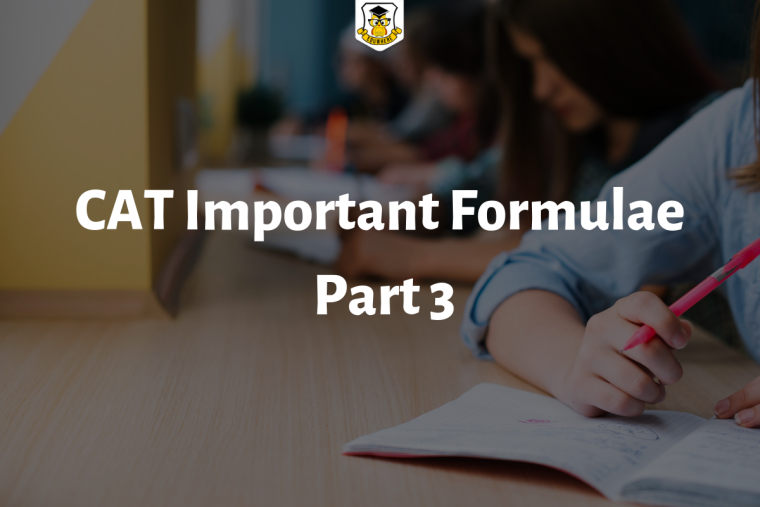## CAT Important Formulae: Part 3Candidates preparing for the CAT 2019 examination can expect 2- 3 questions from Permutation & Combination topic in the Quantitative Section. Here are some important formulae related to Permutation & Combination.

Click here to take online mock tests for CAT 2019 at Eduwhere

Factorial Notation:

Let n be a positive integer. Then, factorial n denoted n! is defined as:

n! = n(n - 1)(n - 2) ... 3.2.1.

Permutations:

The different arrangements of a given number of things by taking some or all at a time, are called permutations.

Number of Permutations:

Number of all permutations of n things, taken r at a time, is given by:

nPr = n(n - 1)(n - 2) ... (n - r + 1) = n!

(n - r)!

Combinations:

Each of the different groups or selections which can be formed by taking some or all of a number of objects is called a combination.

Number of Combinations:

The number of all combinations of n things, taken r at a time is:

nCr = n! = n(n - 1)(n - 2) ... to r factors

(r!)(n - r)! r!

Note:

nCn = 1 and nC0 = 1.

nCr = nC(n - r)

1

1581